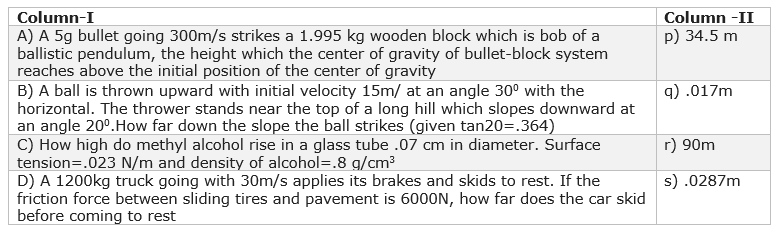Q.1
##### A nearly mass-less rod is pivoted at one end so it can freely swing as a pendulum.Two masses 2 m and m are attached to it at distance a and 3a respectively from the pivot end. The rod is held horizontal and then released. Which of the following is correct?
• The angular acceleration at the instant it is released $\frac {5g}{11a}$
• Moment of inertia of the system about pivot is $11ma^2$correct
• The angular acceleration at the instant when the rod makes an angle $\theta$ with horizontal $\frac {5 g cos \theta }{11a}$correct
• The net torque about the pivot end becomes zero when rod becomes vertical.correct
Q.2
##### A particle is constraint to move along x-axis acted by a force whose potential energy is given by $U=2x^3-15x^2+36x+100$ Choose the correct option
• The force acting on the particle is $6x^2-30x+36$
• If it is slightly displaced from x=3,it will oscillate
• The minimum K.E required at origin to reach at x=1 will be 23 J
• The minimum K.E required at origin to reach at x=2 will be 28 Jcorrect
Q.3
##### Blocks A and B of mass 3 Kg and 2 Kg respectively ,placed on the horizontal smooth surface.They are connected by a mass-less spring. Block A is given a kicks which imparts a velocity of 10 m/s to its towards B
• The velocity of the Center of mass of the system remains constant through out the motion and its value is 6 m/s
• The velocity of block B in Center of mass frame at t=0 is -6 m/s
• The velocity of block A in Center of mass frame at t=0 is 4 m/s
• Total energy of the system is 150 Jcorrect
Q.4
##### Match the columns Column-I Column-II A) A particle moving with constant velocity on a horizontal plane p) P=constant B) Motions of object under central forces q) L=constant C) A object fired from a gun explodes in air r) K.E=constant D) A object in Uniform circular motion in horizontal plane s) PE is constant
• A(p,r,s),B(q),C(p,q),D(q,r,s)
• A(p,q,r,s),B(q,r),C(p),D(q,r,s)
• A(p,q,r,s),B(p),C(p),D(q,r,s)
• A(p,q,r,s),B(q),C(p),D(q,r,s)
Q.5
##### Match the column• A(s),B(p),C(r),D(q)
• A(p),B(s),C(q),D(r)
• A(s),B(q),C(p),D(r)
• A(s),B(p),C(q),D(r)
Q.6
• 1
• 2
• 4
• 3
Q.7
• 2 cm
• 3 cm
• 4 cm
• 5 cm
Q.8
• 4
• 8
• 10
• 6
Q.9
• 1
• 2
• 3
• 4
Q.10
• 4
• 1
• 3
• 5
Q.11
##### Centrifugal force is an inertial force when considered by
• An observer who is moving with the particle that experience the force
• an outside observer
• An observer at the center of the circular motion
• None of the above
Q.12
##### Choose the correct statement
• Two important differences between viscous forces and frictional forces are that viscous force depends upon the temperature and also velocity profile for the liquid where as frictional forces is usually independent of such items
• The terminal velocity of a spherical body falling in a fluid depends on the diameter and density of the body as well the density and viscosity of the fluidcorrect
• M drops of a fluid ,each with surface energy k,join to form a single drop,the amount of energy released in the process is K(M - M^{2/3})correct
• Tiny insects can float and walk on the surface of water due to both buoyancy and surface tensioncorrect
Q.13
##### A pendulum is constructed from two identical uniform rods a and b each of length L and mass M,connected at right angles to form a T by joining the center of rod a to one end of the rod b. The T pendulum is then suspended from free end of rod b and pendulum swings in the plane of T The moment of inertia of T about the axis of rotation
• $\frac {11ML^2}{12}$
• $\frac {7ML^2}{12}$
• $\frac {17ML^2}{18}$
• $\frac {17ML^2}{12}$
Q.14
##### The potential energy change of the T when it is rotated by angle $\theta$ from vertical position
• $\frac {3Mg}{L}(1-cos \theta)$
• $\frac {3Mg}{L}(1-sin \theta)$
• $\frac {4Mg}{L}(1-sin \theta)$
• $\frac {2Mg}{L}(1-sin \theta)$
Q.15
##### For small angle with vertical position,The T makes SHM,the angular frequency
• $\sqrt { \frac {2g}{17L}}$
• $\sqrt { \frac {17g}{19L}}$
• $\sqrt { \frac {12g}{11L}}$
• $\sqrt { \frac {18g}{17L}}$
Q.16
##### A vertical cylinder of cross-sectional area S contains one mole of ideal monoatomic gas under a piston of Mass M. At t=0, an energy source which transfer Q per unit time to gas is switched on under the piston. The gas pressure remains constant and equal to PAnd the gas is thermally insulated. What will be the established velocity of the piston?
• $v=\frac {2}{5} \frac { Q}{(P_0 A+Mg)}$
• $v=\frac {52}{52} \frac { Q}{(P_0 A+Mg)}$
• $v= \frac { Q}{(P_0 A+Mg)}$
• None of the above
Q.17
##### Link Comprehension Type Use this to solve the Question 2 and 3 An object moves along a circular path of radius R with deceleration. At any moment, the magnitudes of tangential and normal acceleration are same. The object starts with velocity v0 Find the velocity v of the object as function of distance s covered by it
• $v=v_0 e^(-R/s)$
• $v=v_0 e^(-2s/R)$
• $v=v_0 e^(-s/2R)$
• $v=v_0 e^(-s/R)$
Q.18
##### The total acceleration as a function of distance s covered
• $a=\frac {\sqrt {2} v_0^2 e^(-2s/R)}{R}$
• $a=\frac {v_0^2 e^(-2s/R)}{R}$
Q.20
• 4 V,12 V
• 2 V ,14 V
• 8 V ,16 V
• 122V,778V